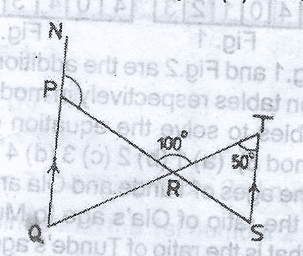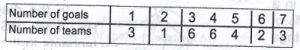# Mathematics 2017 Past Questions | WAEC

Study the following Mathematics past questions and answers for JAMBWAEC  NECO and Post-JAMB. Get prepared with official past questions and answers for upcoming examinations.

41.

 Marks 0 1 2 3 4 5 Frequency 7 4 18 12 8 11

The table gives the distribution of marks obtained by a number of pupils in a class test. Using this information, Find the median of the distribution

• A. 4
• B. 3
• C. 1
• D. 2

42.

 Marks 0 1 2 3 4 5 Frequency 7 4 18 12 8 11

The table gives the distribution of marks obtained by a number of pupils in a class test. Using this information, find the first quartile

• A. 1.0
• B. 1.5
• C. 2.0
• D. 2.5

43. In a class of 45 students, 28 offer chemistry and 25 offer Biology. If each student offers at least one of the two subjects, calculate the probability that a student selected at random from the class the class offers chemistry only.

• A. 2/9
• B. 4/9
• C. 5/9
• D. 7/9

44.In the diagram, NQ//TS, <RTS = 50o${}^{o}$ and <PRT = 100º. Find the value of <NPR

• A. 110${o}^{}$
• B. 130${o}^{}$
• C. 140${o}^{}$
• D. 150º

45. A stationary boat is observed from a height of 100m. If the horizontal distance between the observer and the boat is 80m, calculate, correct to two decimal places, the angles of depression of the boat from point of observation

• A. 36.87º
• B. 39.70º
• C. 51.34º
• D. 53.13o

46.  The diagonal of a square is 60 cm. Calculate its peremeter

• A. 20$$\sqrt{2}$$
• B. 40$$\sqrt{2}$$
• C. 90$$\sqrt{2}$$
• D. 120$$\sqrt{2}$$

47. Find the value of m in the diagram

• A. 72º
• B. 68º
• C. 44º
• D. 34º

48. The graph of y =  $a{\mathrm{x²}}^{}+bx+c$is shown on the diagram. Find the minimum value of y

• A. -2, 0
• B. -2, 1
• C. -2, 3
• D. -2, 5

49.  In the diagram, PR is a diameter of the circle RSP, RP is produced to T and TS is a tangent to the circle at S. If < PRS = 24º, calculate the value of < STR

• A. 24º
• B. 42º
• C. 48º
• D. 66º

50.The table shows the distribution of goals scored by 25 teams in a football competition. Calculate the probability that a team selected at random scored at most 3 goals.

• A. $\frac{3/}{25}$
• B. $\frac{1/}{5}$
• C. $\frac{6/}{25}$
• D. 2/5
Subscribe
Notify of